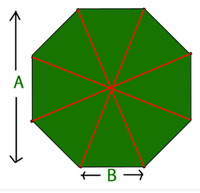# Area of an Octagon Formula

• Last Updated : 17 Jan, 2022

Polygon is a closed, connected shape made of straight lines. It may be a flat or a plane figure spanned across two dimensions. The word Polygon is made up of 2 words first Poly means “many” and gons means “sides”. A polygon is created by using straight-line segments that are end to end connected with each other, and these line segments are known as sides of the polygon and the point is known as the vertex of the polygon. If a shape does not contain sides and angles then they are not a polygon-like circle. Some of the polygons are Square, Triangle, Pentagon, Hexagon, Octagon, etc.

A regular octagon is a closed figure with sides of the same length and internal angles of the same size. It has eight lines of reflective symmetry and rotational symmetry of order 8. The internal angle at each vertex of a regular octagon is 135°. The central angle is 45°.

Structure of an OctagonProperties of an Octagon

1. An Octagon has eight sides and eight angles.
2. All Lengths of all the sides and the measurement of all the angles in an Octagon are equal.
3. The total number of diagonals present in an octagon is 20.
4. The sum of all interior angles is equal to 1080 degrees, with each interior angle measuring 135 degrees.
5. The sum of all exterior angles is equal to 360 degrees, with each exterior angle measuring 45 degrees

Formula for Area of an Octagon

2 × (side)² × (1+√2)

where, side is the side length of the octagon

### Sample Problems

Question 1: Find the Area of an Octagon whose side is 8 cm.

Solution:

We know that side value is 8

So, substitute the value in area formula

=> 2 × (8)² × (1+√2) =>2*64*2.414 => 308.892 cm

Therefore the Area of an Octagon whose side 8 cm is 308.892 cm

Question 2: Find the Area of an Octagon whose side is 34 cm

Solution

We know that side value is 34

So, substitute the value in area formula

=> 2 × (34)² × (1+√2) =>2*1156*2.414 =>5581.1682 cm

Therefore the Area of an Octagon whose side 34 cm is 5581.1682 cm

Question 3: Find the Area of a swimming pool with an Octagon shape with one side 1 cm.

Solution:

We know that side value is 1 cm

So, Substitute the value in area formula

=> 2 × (1)² × (1+√2) =>2*1*2.414 =>4.828 cm

Therefore the Area of an swimming pool with Octagon shape with one side 1 cm is 4.828 cm

Question 4: Find the Area of a 500 gram weight with one side 3.4 cm.

Solution:

We know that side value is 3.4 cm

So, Substitute the value in area formula

=> 2 × (3.4)² × (1+√2) =>2*11.56*2.414 =>55.811 cm

Therefore the Area of an 500 gram weight with one side 3.4 cm is 4.55.811 cm

Question 5: Side of a base tower in octagonal shape is 3.4 cm. What is the area?

Solution:

We know that side value  is  3.4 cm

so, Substitute the value in area formula

=>  2 × (3.4)² × (1+√2)) =>2*11.56*2.414 =>55.811 cm

Therefore the Area of  tower base with one side  3.4 cm is 4.55.811 cm

My Personal Notes arrow_drop_up
Recommended Articles
Page :